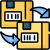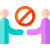-35%

## Solutions to I.E.Irodov’s Problems in General Physics

260.00

• Name of the Textbook: Solutions to I.E.Irodov’s Problems in General Physics
• Edition: v. 1
• Author: Singh A. K.
• Publisher: Singh A. K.
• ISBN: 9788126520763
• Language: english
• Book: This Is Used Books But Condition Is Good Shop With Confidence

• (Save with offers) Pay with (Debit/Credit,Paytm,UPI,Gpay,Phonepe) and get 3% refund in 24 hr.
• ### Services:

•Cash on Delivery: available
•Replace: 5 Days Replacement
•No-Contact Delivery
Category:

## Description

This book is the first of a two volume set providing solutions to Irodov’s Problems in General Physics.

Summary Of The Book

Irodov’s Problems are a set of problems in general physics designed by the Russian physicist I.E. Irodov. They were designed to test students’ understanding of physics concepts and formulas. The problems range from the simple to the highly complex.

Irodov’s book of problems is a prescribed study material for many competitive science exams. It is part of the basic preparation for the IIT-JEE exam. These problems are considered to be the most testing and intricate, and some of them combine multiple concepts in physics, making them quite challenging.

This book is the first of a two volume set providing detailed solutions to all the 1878 problems devised by Irodov. The books are divided into sections that match the divisions in the original book of problems by Irodov.

This book, Volume 1, contains physical fundamentals of mechanics, thermodynamics and molecular physics, and electrodynamics. Each part is divided into smaller chapters dealing with all the related problems in subtopics.

The first part covers problems in kinematics, the fundamental equation of dynamics, universal gravitation, dynamics of a solid body, elastic deformation of a solid body, hydrodynamics, and relativistic mechanics.

The problems in the second part are in the topics equations of the gas state processes, the first law of thermodynamics, heat capacity, kinetic theory of gases, Boltzmann’s law and Maxwell’s distribution, liquids, capillary effects, phase transformations, and transport phenomena.

The third part problems cover constant electric field in vacuum, conductors and dielectrics in an electric field, electric capacitance, energy of an electric field, electric current, constant magnetic field, magnetics, electromagnetic induction, Maxwell’s equations, and motion of charged particles in electric and magnetic fields.

The book covers the salient features in each topic. It provides comprehensive solutions for all problems with alternative solutions for around 30% of these problems. Explanatory diagrams accompany 80% of the problems, and the solutions are given in SI units for accuracy.